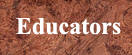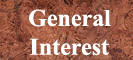Cybrary Man's Educational Web Sites
The internet catalogue for students, teachers, administrators & parents.

Over 20,000 relevant links personally selected by an educator/author with over 30 years of experience.

 Educators         Parents        Students       General     Home

# Number Tricks

Mental Shortcuts or Mentally Efficient Maths

For these activities have the students use a pencil or pen and paper to write down each step.
PROBLEM “A”

Have student choose any number and to add 9 to it. (It would be easier if they pick small numbers to do this.)

Have student multiply this number by 2.

Subtract 4.

Divide the remainder by 2.

Subtract the number first chosen.

PROBLEM “B”

Tell a student to choose any number and add 5 to it.

Multiply this result by 3.

Subtract 9.

Divide the remainder by 3.

Subtract the number that was first selected.

Now, you can give the student the result.

PROBLEM “C”

Ask a student to select two numbers, each of which is less than 10.

Tell the student to choose either of the numbers and to multiply it by 5.

Tell the student to add 7 to this result.

Multiply the resulting sum by 2.

Add it to the other number that was first selected.

Have the student tell you the result.

Now you can tell the student the two numbers that were first chosen and also the one which was multiplied by 5.

PROBLEM “A”
The result will always be “7” no matter what number was chosen.

PROBLEM “B”
The result will always be 2.

PROBLEM “C”
From whatever result the student gives you, subtract 14. You will then have a number of two figures. The two figures of this number are the two numbers which the person selected and the figure in “ten’s” place, that is the left-hand figure, is the one which the student multiplied by 5.

For example, if the student says, “My result is 88”, you subtract 14 from 88. The result is 74. The two numbers which the student chose were, therefore, 7 and 4, and, since 7 is the ten’s figure of 74, it is the number which he multiplied by 5.

Finding Someone’s Age

Ask the person to multiply the first number of his or her age by 5.

Now tell them to double this figure.

Finally, have the person add the second number of his or her age to the figure and have them tell you their answer.

Deduct 6 and you will have their age.

Now multiply it by 1,443

What do you get?

Birthday Numbers and Tricks

Mental Math

Math pages

JN19